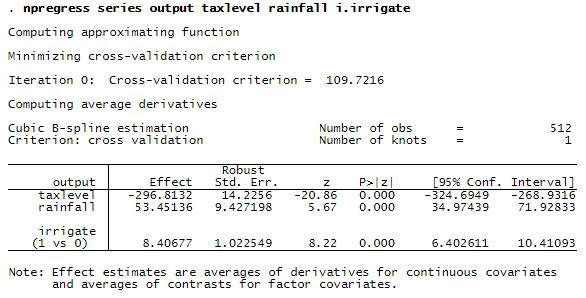NONPARAMETRIC SERIES REGRESSION

Nonparametric series regression (NPSR) estimates mean outcomes for a given set of covariates, just like linear regression. Unlike linear regression, NPSR is agnostic about the functional form of the outcome in terms of the covariates, which means that NPSR is not subject to misspecification error.

In NPSR, you specify the dependent variable and its determinants. NPSR determines the functional form. If you type

. npregress series y x1 x2 x3



you are specifying that

y=g(x1,x2,x3)+ϵy=g(x1,x2,x3)+ϵ

You are placing no functional-form restrictions on g()g()g()g() is not required to be linear, although it could be.

g(x1,x2,x3)=β1x1+β2x2+β3x2g(x1,x2,x3)=β1×1+β2×2+β3×2

g()g() is not required to be linear in the parameters, although it could be.

g(x1,x2,x3)=β1x1+β2x22+β3x31x2+β4x3g(x1,x2,x3)=β1×1+β2×22+β3x13x2+β4×3

Or g()g() could be

g(x1,x2,x3)=β1xβ21+g(x1,x2,x3)=β1×1β2+ cos(x2x3)+ϵ(x2x3)+ϵ

Or g()g() could be anything else you can imagine.

The jargon for this is that g()g() is fully nonparametric.

What you specify does not have to be fully nonparametric. You can impose structure.

Type

. npregress series y x1 x2 x3, nointeract(x3)



and you are specifying

y=g1(x1,x2)+g2(x3)+ϵy=g1(x1,x2)+g2(x3)+ϵ

Type

. npregress series y x1 x2 x3, nointeract(x2 x3)



and you are specifying

y=g1(x1)+g2(x2)+g3(x3)+ϵy=g1(x1)+g2(x2)+g3(x3)+ϵ

Type

. npregress series y x1 x2, known(x3)



and you are specifying

y=g1(x1,x2)+β3x3+ϵy=g1(x1,x2)+β3×3+ϵ

You specify how general—how nonparametric—the model is that you want to fit.

The fitted model is not returned in algebraic form. In fact, the function is never even found in algebraic form. It is approximated by a series, and you can choose polynomial series, natural spline series, or a B-spline series. npregress series reports

average marginal effects for continuous covariates

contrasts for discrete covariates

npregress series needs more observations than linear regression to produce consistent estimates, and the number of observations required grows with the number of covariates and the complexity of g()g().

Let’s see it work

We have fictional data on wine output from 512 wine-producing counties around the world. output will be our dependent variable. We believe output is affected by

 taxlevel taxes on wine production rainfall rainfall in mm/hour irrigate whether winery irrigates

Our main interest is to see how tax levels affect wine yield, and we include rainfall and irrigate as controls so that the effect of taxlevel is correctly measured.

We start by fitting the model.The output reports effects of -297, 53, and 8.4 for taxlevelrainfall, and irrigate

Start with the -297. taxlevel is a continuous variable, so -297 is a “average marginal effect”, meaning it is the average derivative of output with respect to taxlevel. Said differently, the marginal effect is what economists would call the average marginal effect of taxes on output. Higher taxes result in lower output.

Now consider the 53, which is also an average marginal effect because rainfall is a continuous variable. Higher rainfall increases wine output.

Finally, there is the 8.4, which is a contrast because irrigate is a factor (dummy) variable. irrigate is 1 if the wine grower irrigates and 0 otherwise. The contrast of 8.4 is the average effect for a discrete change. It is the difference of what the mean output would be if all producers irrigated and what the mean output would be if no producers irrigated. 8.4 means a positive treatment effect of irrigation.

Do these estimated effects answer your research question? They might, but if they do not, we can obtain whatever estimated effects we need using Stata’s margins command. If we need to explore the effects of various tax levels, say between 11 and 29 percent, we can type

.  margins, at(taxlevel=(.11(.03).29))

(output omitted)


It produces a table of effects and standard errors that we omitted because we want to show the result graphically, which we do simply by typing marginsplot after producing a table using margins.

. marginsplotThe effect of taxes is not linear.

You are not restricted to exploring the function one variable at a time. You could investigate the mean output for different levels of taxes and irrigation by typing

. margins irrigate, at(taxlevel=(.11(.03).29))



You could investigate mean output for different levels of taxes and irrigation and rainfall by typing

. margins irrigate, at(taxlevel=(.11(.03).29)) at(rainfall=(.01(.05).33))



Take a minute to appreciate this. We believe that wine output is a function of taxes, rainfall, and irrigation, but we do not know the function. We can nonetheless fit an approximation to the unknown function and explore it to gain statistical insight using npregress seriesmargins, and marginsplot